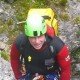# The American Death Triangle in the real world## The American Death Triangle in the real world

Recently (Feb 2018) I wrote a blog on the forces involved in the American Death Triangle. I questioned if there were some occasions we can use this style of rigging. Note: I would recommend reading this first article before diving into this one: American Death Triangle and driving on the wrong side of the road

My conclusions for side-by-side ring bolts: threading the rope through the rings and abseiling on double ropes keep forces acceptable, so long as you attach (ALTITUDE) at a further distance than the bolts are apart (BASE). With this attachment, you are putting a factor of less than 1 (100%) on each anchor, and the angle formed by the two ropes (APEX ANGLE) is less than 60 degrees.### What about the real world?

Would the theory hold up in the real world where rope friction around the bolts might affect the forces? Would the abseiler, with the usual bounce and swing on the rope, change the result? We were back up at the Nelson Speleological Group (New Zealand) SRT tower so decided to test the theory.

### The setup

• We had two Rock Exotica Enforcer Load Cells clipped into two ring bolt anchors.
• We threaded a 10mm Kordas Iris semi-static through the load cell swivel eyes.
• When we pulled the ropes together by hand, the swivel eyes were around 210mm apart.
• We did two abseils each – one with the swivel eyes at head height (attached around 400mm below), and one we climbed higher so that we were closer (attached around 230mm below).
• We abseiled with what we considered normal loading, without undue bounce or swing however not taking any additional care because of the tests.

### Test 1 – Apex angle 30 degrees

#### Theory

In theory, the force on each anchor is a factor of 0.8 (80%) of the load. Note: The LHS/RHS was from the abseilers perspective while they were standing on the SRT tower platform facing the ropes.#### Actuals

 Mass kg Convert to kN LHS kN LHS factor* RHS kN RHS factor* Abseiler 1 95 0.93 0.80 0.86 0.74 0.80 Abseiler 2 90 0.88 0.74 0.84 0.70 0.80 Average 92.50 0.91 0.77 0.85 0.72 0.80

#### Comparison actuals vs theory

 LHS kN LHS factor* RHS kN RHS factor* Actuals 0.77 0.85 0.72 0.80 Theory 0.72+ 0.80 0.72+ 0.80 Difference 0.05 0.05 0.00 0.00

* Factor: Proportion applied where the load is equal to 1. Rather than use percentages, we convert to factors to make our life easier for multiplication. The factor is the same value as percentages but represented as a decimal. e.g., a factor of 0.8 is the same as 80%, a factor of 1 is 100%, a factor of 1.3 is 130%, etc.

+ Theory kN: The average kN of the abseilers (e.g., 0.91kN) times the theoretical factor (e.g., 0.80)

### Test 2 – Apex angle 50 degrees

#### Theory

In theory, the force on each anchor is a factor of 1.0 (100%) of the load.#### Actuals

 Mass kg Convert to kN LHS kN LHS factor* RHS kN RHS factor* Abseiler 1 95 0.93 0.78 0.84 0.94 1.01 Abseiler 2 90 0.88 0.88 1.00 0.92 1.05 Average 92.50 0.91 0.83 0.92 0.93 1.03

#### Comparison actuals vs theory

 LHS kN LHS factor* RHS kN RHS factor* Actuals 0.83 0.92 0.93 1.03 Theory 0.91+ 1.00 0.91+ 1.00 Difference -0.08 -0.08 0.02 0.03

### Conclusions and observations

1. The theory and real world did align very closely.
2. The further away you attach the less load you put on the anchors. Conversely the closer you attach the more load you put on the anchors.
3. We found it easy to load one anchor more than the other, i.e., offset anchor alignment. I think this was partly due to the load cells being free floating rather than the rope threaded through a fixed ring bolt anchor attached to a rock wall. However, this was not a significant issue to be a concern.
4. The same advice applies from the previous article:
• Rule of thumb: Attach to the double rope at a distance equal to or greater than the bolts are apart. For example, if the bolts were 200mm apart, you need to attach a minimum of 200mm below.
• The smaller the APEX angle, the less the force will be on the anchors. So, make the angle less by attaching further away from the side-by-side bolts.

Note: We only did a small number of tests (2) with each setup. More tests would give more valid results.

Check the previous article out here: American Death Triangle and driving on the wrong side of the road

Here is a video we made of the testing so you know it’s real. Remember to subscribe to our YouTube channel.

from the team at Over the Edge Rescue

Grant